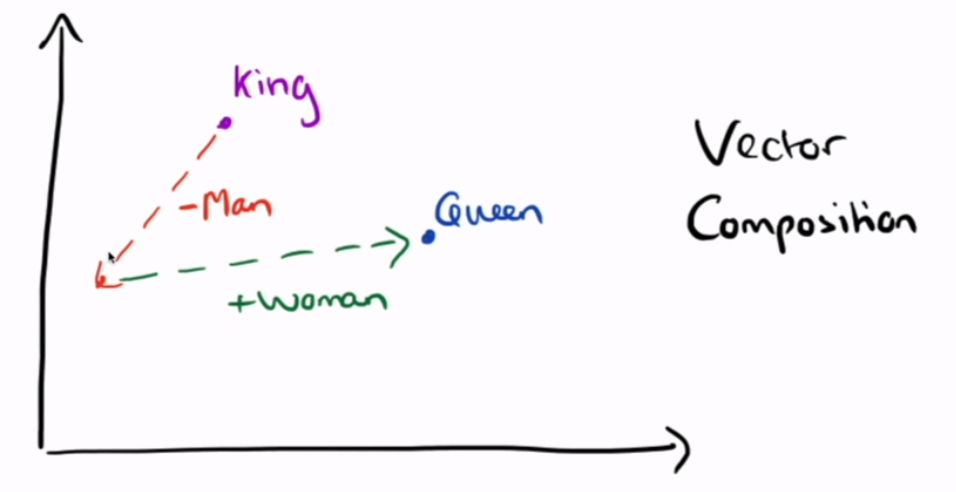# [XCS224N] Lecture 1 – Introduction and Word Vectors

### Course intro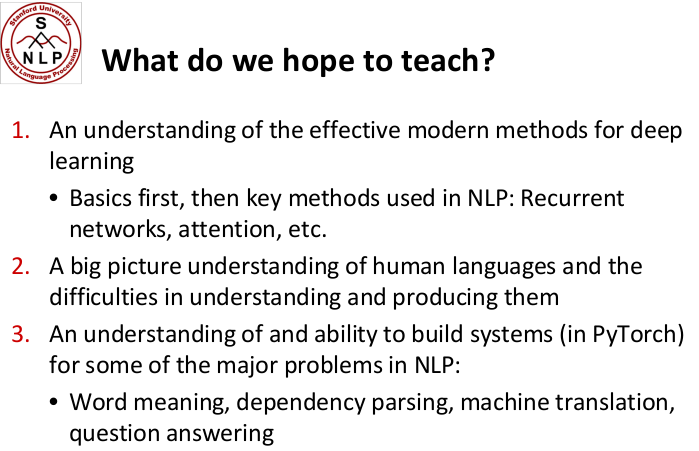### Word Meaning and Representation

denotational semantics

wordnet (nltk): word meanings, synonym, relationships, hierarchical

pb: missing nuance, missing new meanings, required human labor, can't compute word similarity

• each words are discrete symbols — "localist representation"
• use one-hot vectors for encoding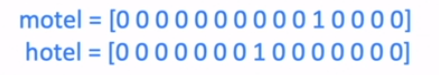• pbs with one-hot vecotrs:
• large dimension size
• each two words are orthogonal, no relationships between them

distributional semantics: A word’s meaning is given by the words that frequently appear close-by. ⇒ Use the many contexts of w to build up a representation of w.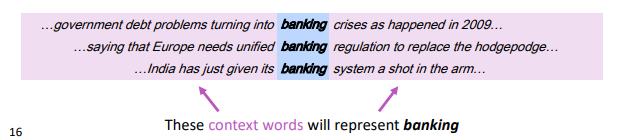"distributed representation": use dense vectors for each word, so that it is similar to vectors of words that appear in similar contexts.

### Word2Vec Introduction

word2vec algo: for learning word embeddings.

#### idea

• each word from the (fixed) vocab has a vector v — start with rand vectors
• for each center word `c` and context(outside) word `o` :
• use `sim(c,o)` to compute `P(o|c)` or `P(c|o)` .
• update the vecs to maximize the probability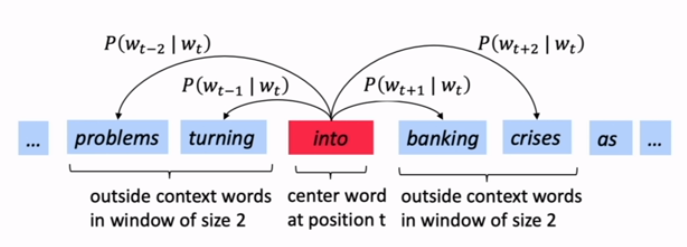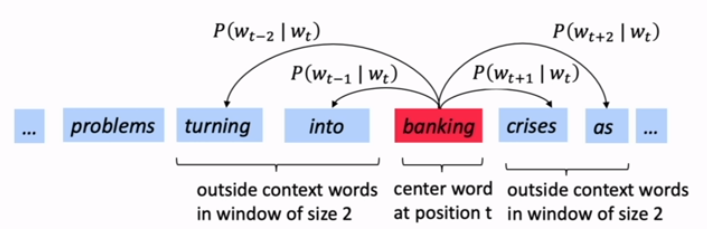#### likelihood

Likelihood := product of all predicted probabilities for all window words (fixed window sz= `m` ), for all positions `t` .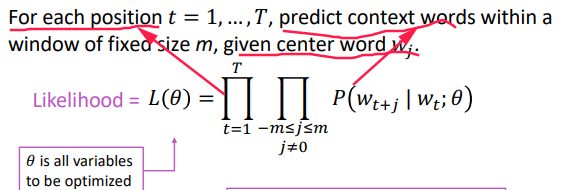#### Loss function

⇒ take negative log likelihood as loss function: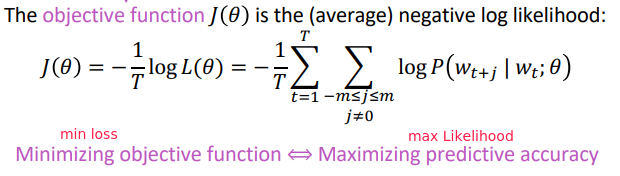#### Prediction function

⇒ probability prediction `P(o|c)` is a function of the word vectors:

• we use two vectors per word:
• use `v_w` when word w is the center word
• use `u_w` when w is context word.
• proba = softmax of the dot products `dot(v,u)` for all context word u in Vocab.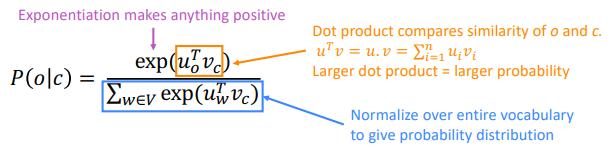•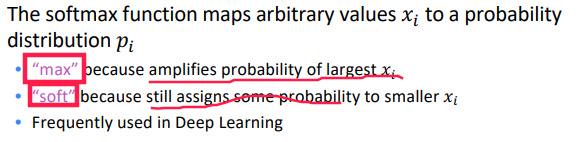#### optimization

`θ` :=all parameters in the model, i.e. `2*V` vectors (u/v), each of dimension `d` .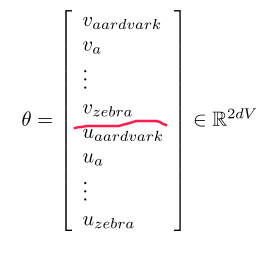gradient descent: compute all gradients of `θ` w.r.t.loss function `J(θ)` . I.e.compute dJ(θ)/dθ, which is `2*d*V` dimension.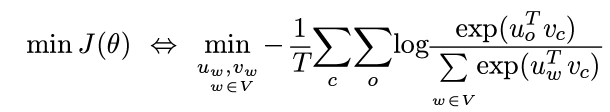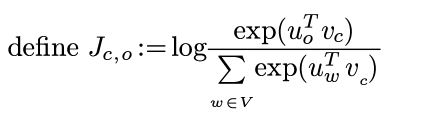using chain rule and multi-var derivative: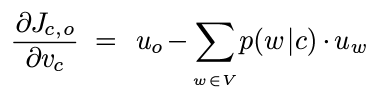⇒ slope of the vector `v_c` w.r.t. `J_c,o` equals the current vector `u_o` minus the weighted average of context word `u_w` , i.e."expected context word".

## Gensim Word Vector Visualization

gensim: word similarity package

word composition